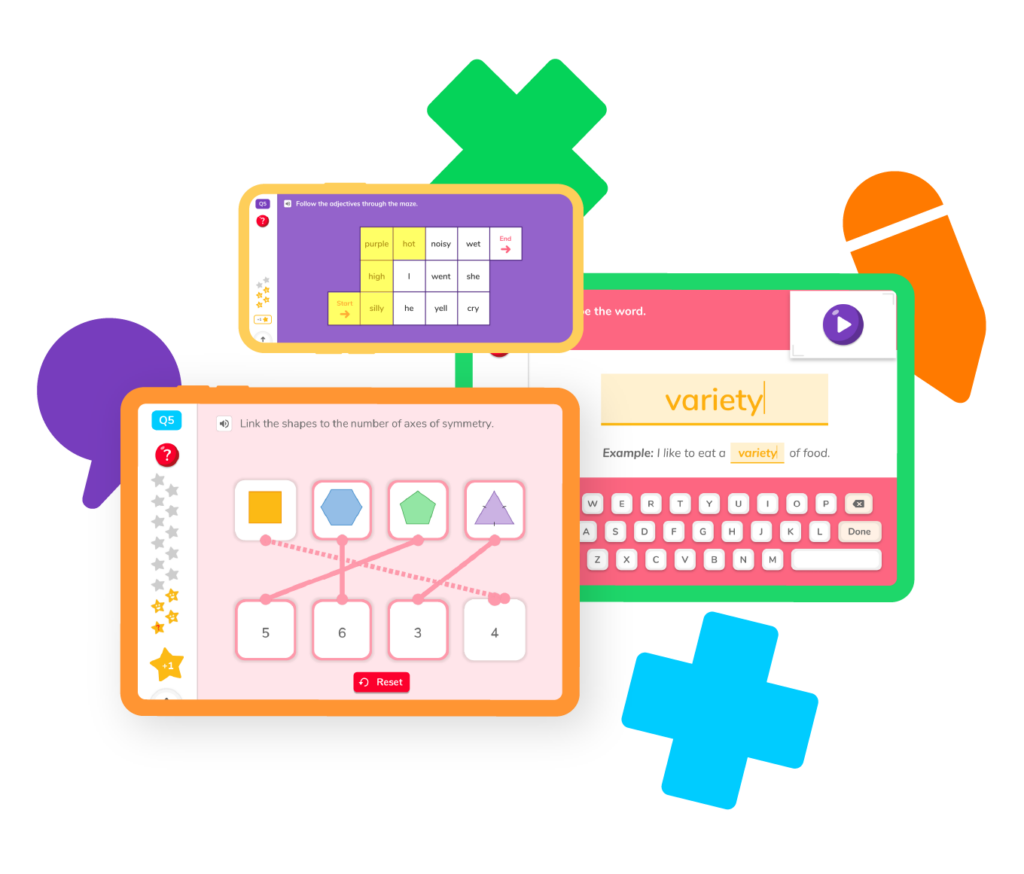# Learning Subtraction

When it comes to subtraction, less is more! Well, maybe not, but it does mean taking one number away from another number to get a whole new result. As one of the core mathematical concepts, subtraction is the key to understanding and succeeding in math. Let’s explore the subtraction skills you need to master this important concept!## Subtraction resourcesSubtraction starts simple but builds to more complicated operations as you go. Practice your skills: taking away certain items and counting what’s left, and later, subtracting decimals and fractions.

## Take away shapes and pictures

Practice taking away items and counting the items left to understand subtraction.

## Find the difference

Solve basic subtraction problems to find the difference between two numbers.

## Subtraction with regrouping

Learn how to use regrouping to subtract multi-digit numbers.

## Borrowing

Explore how to borrow numbers from certain place values to find the difference.

## Subtracting fractions

Discover how to subtract 1/3 from 1/2.

## Subtracting decimals

Practice subtracting decimals to find the correct answer.

## Take away shapes and pictures

Practice taking away items and counting the items left to understand subtraction.

## Find the difference

Solve basic subtraction problems to find the difference between two numbers.

## Subtraction with regrouping

Learn how to use regrouping to subtract multi-digit numbers.

## Borrowing

Explore how to borrow numbers from certain place values to find the difference.

## Subtracting fractions

Discover how to subtract 1/3 from 1/2.

## Subtracting decimals

Practice subtracting decimals to find the correct answer.It takes practice and time to master subtraction, but we know you can do it! We put together a list of some of the most frequently asked questions about learning subtraction.

### What terms do I need to know to understand subtraction?

There are a few vocabulary words you will need to know to understand subtraction. These terms include:

• The minuend – The number that you are subtracting from
• The subtrahend – The number you are going to subtract from the minuend
• The difference – The result of subtracting the subtrahend from the minuend

### What strategies can I use to subtract successfully?

The best subtraction strategy depends on the problem you are trying to solve. Strategies to find the difference between two numbers include regrouping, borrowing, and using number lines.

### How is subtraction related to addition?

Subtraction and addition are inverse operations. This means they are opposite operations from one another. Where adding means you are adding to, subtracting means you are taking away. If you have six apples and you add four more, you will have 10 apples. If you have 10 apples and take away four, you will have six apples.

### When will my child start learning subtraction?

Many public schools lay the foundation for subtraction as early as kindergarten by having children take away cubes or blocks and then count how many are left. Your student will start on subtraction in 1st grade and then begin to practice borrowing, regrouping, and more in 2nd grade.Subtraction has three main parts: the minuend, the subtrahend, and the difference. The minuend is the number you start with or have. The subtrahend is the number you take away or subtract. And the difference is the answer you get when you subtract the subtrahend from the minuend!

To subtract in math, you start with two numbers: the minuend (the bigger number) and the subtrahend (the smaller number). You can use a variety of methods to subtract, but one common way is called “counting back” or “taking away.” You begin with the minuend and count back or take away the subtrahend. The answer you get is the difference.

Let’s say you have 8 apples, but you eat 3 of them. If you want to know how many apples you have left, you can use subtraction. In this case, the minuend is 8 (the total number of apples you had) and the subtrahend is 3 (the number of apples you ate). By subtracting 3 from 8, you find the difference: 8 – 3 = 5. So, you have 5 apples left after eating 3 of them.

Remember, subtraction is a way of finding the difference between two numbers or taking away a quantity from a starting amount.# Are you a parent, teacher or student?

Are you a parent or teacher?

## Hi there!

Book a chat with our team